APPLIED INDUSTRIAL CONTROL SOLUTIONS LLC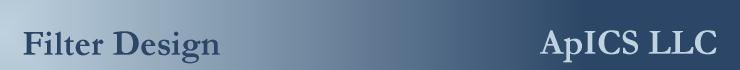Higher Order Analog Butterworth Filter Designs, a Tutorial

Instructions

1. Make sure you have Java turned on in your browser.
2. Enter high and low pass speaker impedances.
3. Enter desired crossover frequency.
4. On the second-order crossover calculator you must select type of crossover.
5. Click on the "calculate" button to get the answers.
• Impedance is the nominal resistance of the speaker (typically 4 Ohms).
• Enter frequency in Hertz (not kHz).
• Capacitor value(s) are given in millionths of a Farad (µF).
• Inductor value(s) are given in thousands of a Henry (mH).
• For the Zobel circuit, enter inductance in Henries (not mH).
• Calculators *

1. First Order Crossover (6db/octave).
2. Second Order Crossover (12db/octave).
3. Third Order Crossover (18db/octave).
4. Fourth Order Crossover (24db/octave).
5. Zobel Circuit (Impedance Stabilization).

First Order (6db/octave) Two-Way Crossover

High Pass Impedance: Ohms
Low Pass Impedance: Ohms
Frequency Hz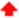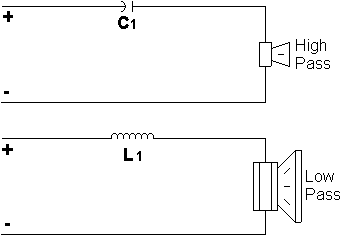C1= µF

L1= mH

 Phase shift on a first-order crossover is 90 degrees.

Second Order (12db/octave) Two-Way Crossover

High Pass Impedance: Ohms
Low Pass Impedance: Ohms
Frequency Hz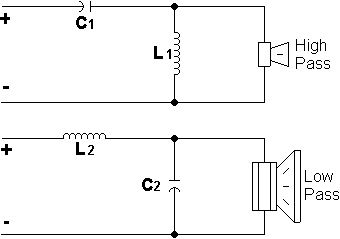C1 = µF

L1 = mH

C2 = µF

L2 = mH

 Linkwitz-Riley crossovers match attenuation slopes so that system response is flat at crossover point. Butterworth crossovers yield to a peak at the crossover frequency. Bessel crossovers have a frequency response between Linkwitz-Riley and Butterworth crossovers. The phase shift on a second-order crossover is 180 degrees (reversed polarity).

Third Order (18db/octave) Two-Way Crossover

High Pass Impedance: Ohms
Low Pass Impedance: Ohms
Frequency Hz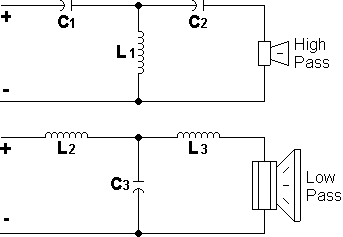C1 = µF
C2 = µF
L1 = mH

L2 = mH
L3 = mH
C3 = µF

 Phase shift on a third-order crossover is 270 degrees (-90 degrees).

Fourth order (24dB/octave) Two-Way Crossover

High Pass Impedance: Ohms
Low Pass Impedance: Ohms
Frequency Hz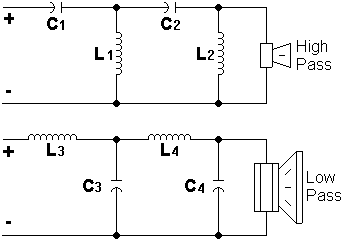C1 = µF
C2 = µF
L1 = mH
L2 = mH

C3 = µF
C4 = µF
L3 = mH
L4 = mH

 The phase shift on a fourth-order crossover is 360 degrees = 0 degrees (no phase shift).

Zobel Circuit (Impedance Stabilization)

DC resistance (Re): Ohms
Inductive Equivalent (Le): Henries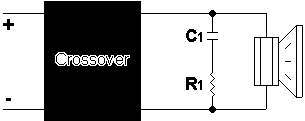C1= µF

R1= Ohms

 Even though speakers are rated at a certain "resistance" (i.e. 4 Ohms), the actual impedance varies with frequency (speakers have inductance). To compensate for the non-linearity of speakers (on mainly subwoofers), Zobel circuits are used. Re is the DC resistance of the woofer (can be measured with an ohmmeter) Le (or Lces) is the electrical inductive equivalent of the driver.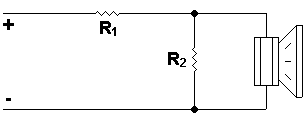R1 = Ohms